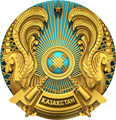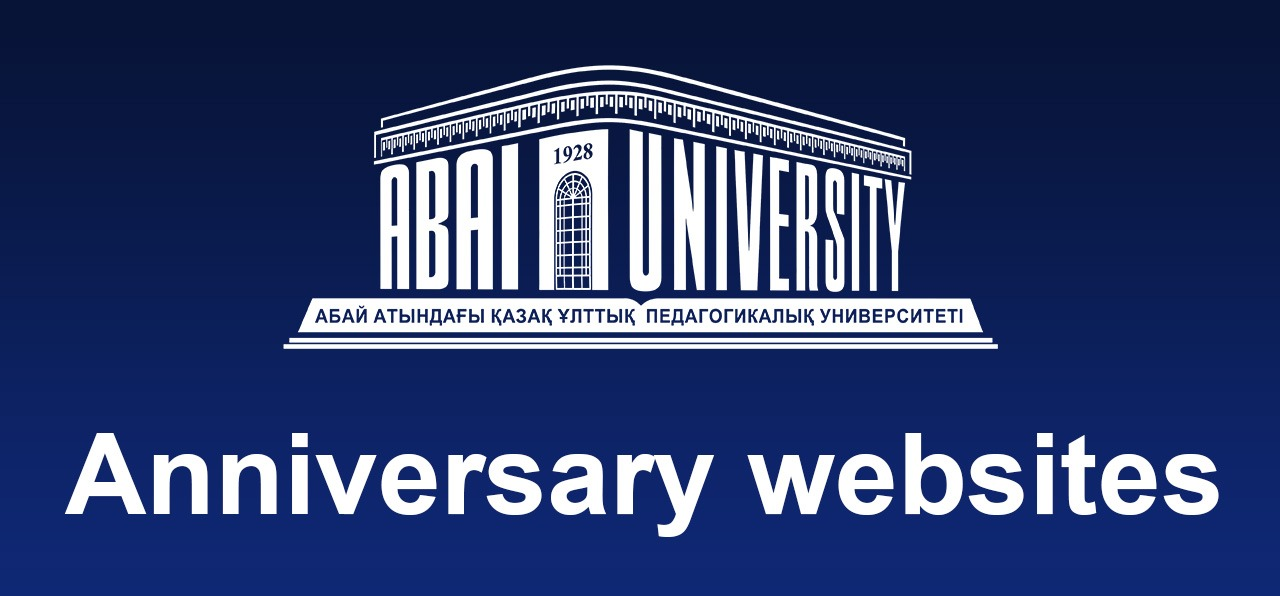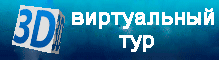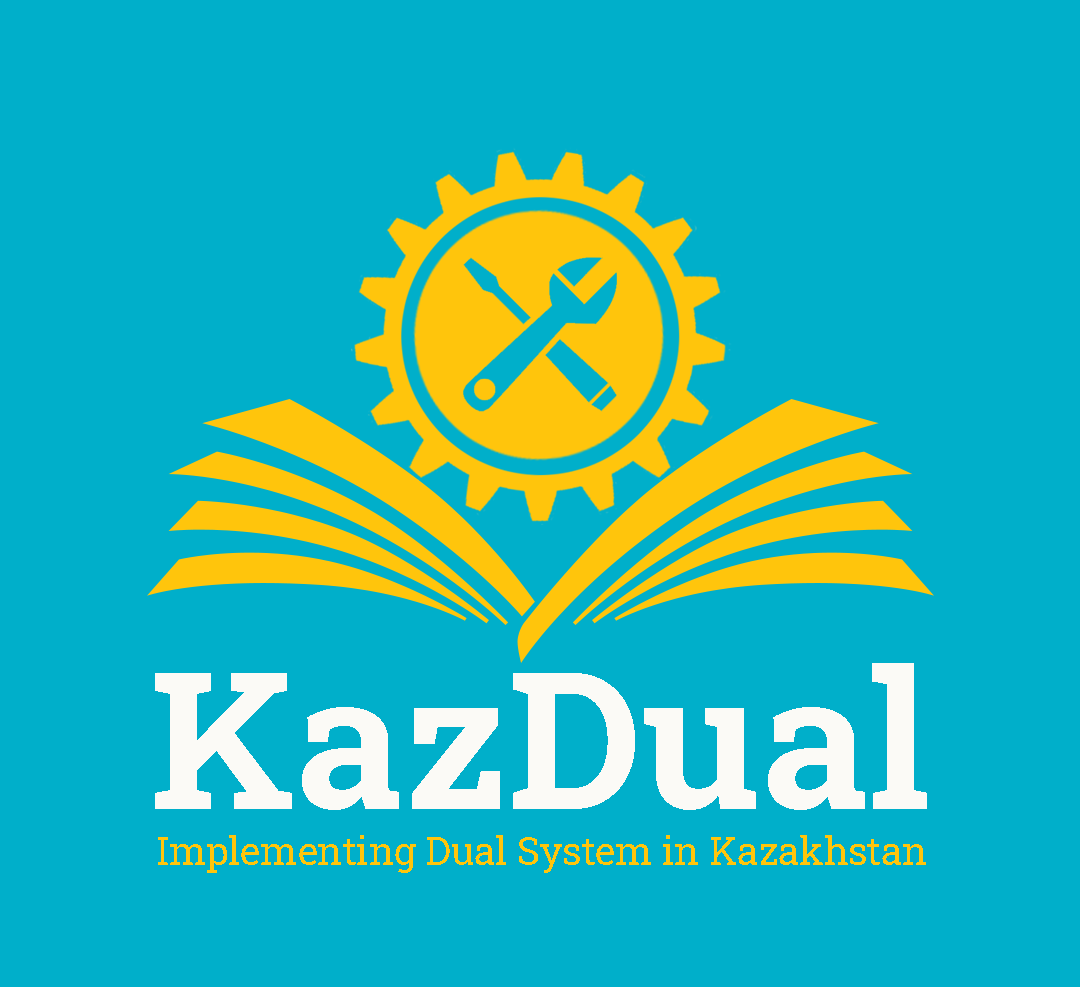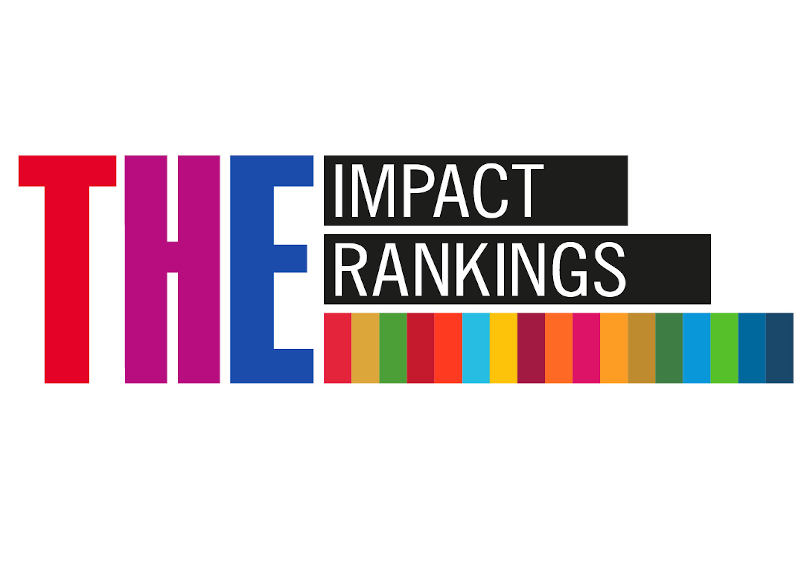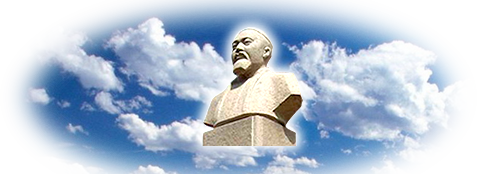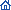Главная / Berdyshev Abdumaulen Suleimenovich Berdyshev Abdumaulen SuleimenovichAbdumauvlen Berdyshev is doctor ofphysical and mathematical sciences. In 1985 he defended his thesis, and in 2000 - his doctoral dissertation. On "Spectral problems in the theory of boundary value problems for equations of mixed and mixed-composite types" (01.01.02 Differential Equations). Professor (2006).Research interests: partialdifferential equations, local and nonlocal boundary value problems for nonclassical equations of mathematical physics, equations of mixed and mixed-composite types, mathematical modeling.He is currently one ofleading experts in the field of the theory of local and non-local problems for differential equations of mixed and mixed-composite types, known in the foreign scientific mathematical communities.He is actively engaged in research and educational work, manages Bachelor, Master and PhD student`s scientific research. He is the chief editor of the journal "Bulletin of Kazakh National Pedagogical University. Series of physical and mathematical sciences "and a member of the editorial board of a number of foreign scientific journals.Scientific works: has a copyright certificate (2015), 167 scientific articles published and 1 monograph "Boundary problems and their spectral properties for equations of mixed parabolic-hyperbolic and mixed-composite type". A.S.Berdyshev obtained significant mathematical results, which were published in such prestigious journals as «Reports of RAS», «Siberian math journal», «Differential equations», «Nonlinear analysis: Theory, Methods & Applications», «Boundary value problems», «Complex Variables and Elliptic Equations», «Acta Mathematica Scienitia», «Applied Mathematical Modelling», «Results in Mathematics», «Electronic Journal of Differential Equations», «Open Computer Science», «Mathematical Methods in the Applied Sciences», «Computers & Mathematics with Applications», «Open Engineering formerly Central European Journal of Engineering».He is an academic adviser of 4 candidates of physical and mathematical sciences and 1 PhD doctor.Awards: Winner of the Youth Union Prize of Uzbekistan in the field of science and technology (1986). He was awarded by medals named after Altynsarin (2006) and "Honorary Worker of Education of the Republic of Kazakhstan" (2008). He is "The best teacher - 2011"grantwinner. ← Вернутся назад